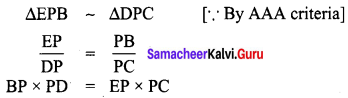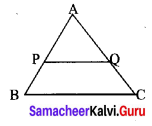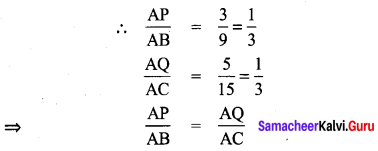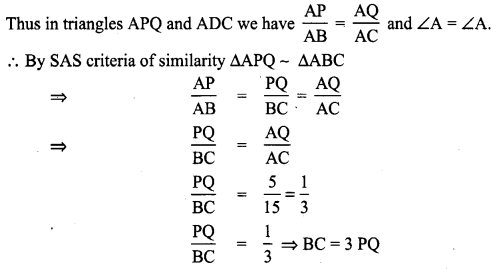Students can Download Maths Chapter 4 Geometry Additional Questions and Answers, Notes Pdf, Samacheer Kalvi 8th Maths Book Solutions Guide Pdf helps you to revise the complete Tamilnadu State Board New Syllabus and score more marks in your examinations.## Tamilnadu Samacheer Kalvi 8th Maths Solutions Term 1 Chapter 4 Geometry Additional Questions

Question 1.
In the given figure if ∠A = ∠C then prove that ∆AOB ~ ∆COD.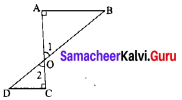Solution:
In triangles ∆AOB and ∆COD
∠A = ∠C (Y given)
∠AOB = ∠COD [∵ Vertically opposite angles]
∠ABO = ∠CDO [Remaining angles of ∆AOB and ∆COD]
∴ ∆AOB ~ ∆COD [∵ AAA similarity]
∵ ∆AOB ~ ∆COD [∵ AAA similarity]

Question 2.
In the figure AB ⊥ BC and DE ⊥ AC prove that ∆ABC ~ ∆AED.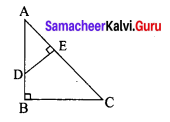Solution:
In triangles ∆ABC and ∆AED
∠ABC = ∠AED = 90°
∠BAC = ∠EAD [Each equal to A]
∠ADE = ∠ACB [∵ Remaining angles]
∴ By AAA criteria of similarity ∆ABC ~ ∆AEDQuestion 1.
In the figure with respect to ABEP and CPD prove that BP × PD = EP × PC.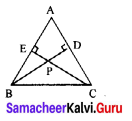Solution:
Proof:
In ∆EPB and ∆DPC
∠PEB = ∠PDC = 90° [given]
∠EPB = ∠DPC [Vertically opposite angles]
∠EPB = ∠PCD [∵ Remaining angles]
Thus,